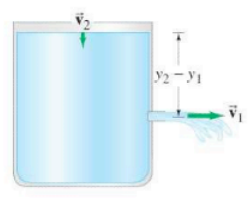×
Get Full Access to Physics: Principles With Applications - 6 Edition - Chapter 10 - Problem 48p
Get Full Access to Physics: Principles With Applications - 6 Edition - Chapter 10 - Problem 48p

×

# (III) In Fig. 10-54, take into account the speed of theISBN: 9780130606204 3

## Solution for problem 48P Chapter 10

Physics: Principles with Applications | 6th Edition

• Textbook Solutions
• 2901 Step-by-step solutions solved by professors and subject experts
• Get 24/7 help from StudySoup virtual teaching assistantsPhysics: Principles with Applications | 6th Edition

4 5 1 430 Reviews
18
4
Problem 48P

(III) In Fig., take into account the speed of the top surface of the tank and show that the speed of fluid leaving the opening at the bottom iswhere, andandare the areas of the opening and of the top surface, respectively. Assumeso that the flow remains nearly steady and laminar.FIGURE 10-54 Problems 48 and 49

Step-by-Step Solution:

Solution 48P:

We have to derive the equation of the velocity of water from the opening at the bottom of the container. This velocity of water is also known as velocity of efflux.

Step 1 of 4

Concept:

Continuity Principle: For the streamlined flow of water, the product of cross sectional areaat any point to the velocityof water at that same point is always constant. Mathematically,…(a)

Bernoulli’s Principle: The sum of the pressure energy, kinetic energy and potential energy for a streamlined system is always constant. Mathematically,…(b)

Step 2 of 4

Step 3 of 4

##### ISBN: 9780130606204

Unlock Textbook Solution